# Computing GCDs over the Gaussian Integers

I wrote up a very simple Perl script for computing GCDs over the Gaussian integers. It comes with no guarantee or proof of correctness. One can sketchily argue something like this however:

Claim: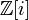$\mathbb{Z}[i]$ is a Euclidean domain, and hence a PID, and hence a UFD.

Consider the norm function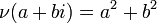$\nu(a + bi) = a^2 + b^2$. We write the standard norm on the complex plane as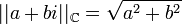$||a+bi||_{\mathbb{C}} = \sqrt{a^2 + b^2}$. We show that for all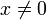$x \neq 0$ and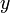$y$ we have,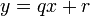$y = qx + r$ where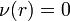$\nu(r) = 0$ or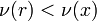$\nu(r) < \nu(x)$. Consider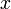$x$ and$y$ as points on the complex plane. Since$x \neq 0$ we have that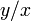$y/x$ is another point in the complex plane. Consider$\mathbb{Z}[i]$ as the points with integer coordinates in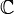$\mathbb{C}$. For any point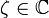$\zeta \in \mathbb{C}$ we can find a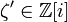$\zeta' \in \mathbb{Z}[i]$ such that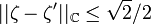$||\zeta - \zeta'||_{\mathbb{C}} \leq \sqrt{2}/2$ by taking the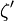$\zeta'$ to be the nearest lattice point to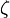$\zeta$. Since any point on the plane is contained in a square whose vertices are lattice points and whose side lengths are one, there must be a lattice point nearer than half the diagonal of the square. We then take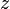$z$ to be the nearest lattice point to$y/x$. We then have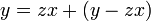$y = zx + (y - zx)$. We compute the norm of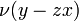$\nu(y - zx)$. We have: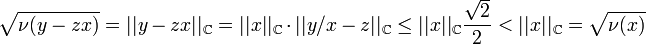$\sqrt{\nu(y - zx)} = ||y - zx||_{\mathbb{C}} = ||x||_{\mathbb{C}} \cdot ||y/x - z||_{\mathbb{C}} \leq ||x||_{\mathbb{C}} \frac{\sqrt{2}}{2} < ||x||_{\mathbb{C}} = \sqrt{\nu(x)}$ It follows that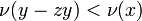$\nu(y - zy) < \nu(x)$. We obtain that$\mathbb{Z}[i]$ is a Euclidean domain. Thus$\mathbb{Z}[i]$ is a PID and hence a UFD by results given in class.

#!/usr/bin/perl
use Math::Complex;

## A Quick hack for computing GCDs of Gaussian integers.

$z2 = 857 + i;$z1 = 255;

sub gcd {
# the Euclidean algorithm

my $x =$_;
my $y =$_;

if ($x *$y == 0) {
print "Done!\n";
} else {
$q = &approx($x/$y);$r = $x -$q*$y; print "($x) = ($q)($y) + ($r)\n"; &gcd($y,$r); } } sub approx { # find the nearest Gaussian integer to a point on the complex plane my$z = $_; my$x = int(Re($z)); my$y = int(Im($z)); if (abs($z - (($x+1) + i*$y) ) < 1/sqrt(2)) {
return ($x+1) + i*$y;
} elsif (abs($z - (($x) + i*($y+1)) ) < 1/sqrt(2)) { return$x + i*($y+1); } elsif (abs($z - (($x+1) + i*($y+1)) ) < 1/sqrt(2)) {
return ($x+1) + i*($y+1);
} else {
return $x + i*$y;
}

}

&gcd($z1,$z2);